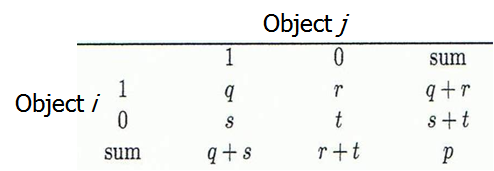# Current Slide

Small screen detected. You are viewing the mobile version of SlideWiki. If you wish to edit slides you will need to use a larger device.

### Proximity Measure for Binary Attributes

• A contingency table for binary data• Distance measure for symmetric binary variables:
$d(i,j)=\frac{r+s}{q+r+s+t}$
• Distance measure for asymmetric binary variables:

$d(i,j)=\frac{r+s}{q+r+s}$

• Jaccard coefficient (similarity measure for asymmetric binary variables):

$sim_{Jaccard}(i,j)=\frac{q}{q+r+s}$

• Note: Jaccard coefficient is the same as “coherence”:

$coherence(i,j)=\frac{sup(i,j)}{sup(i)+sup(j)-sup(i,j)}=\frac{q}{(q+r)(q+s)-q}$

Speaker notes:

## Content Tools

### Sources

There are currently no sources for this slide.